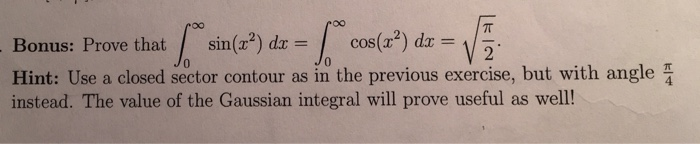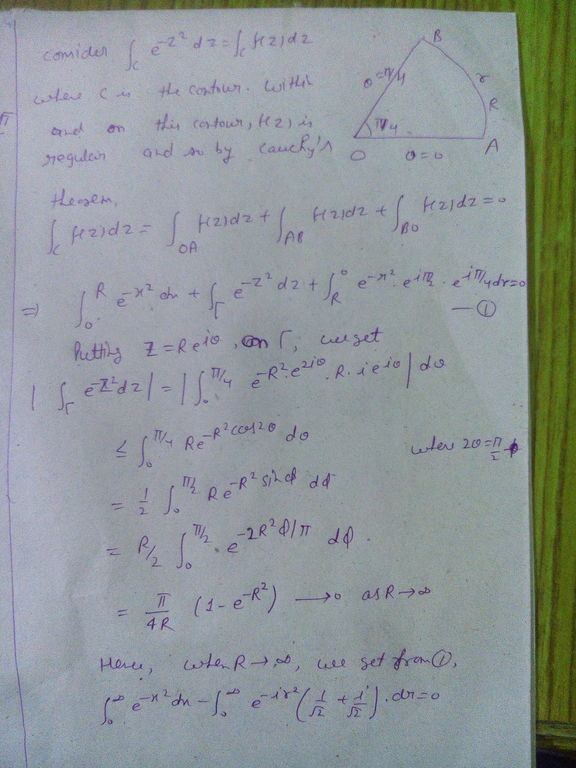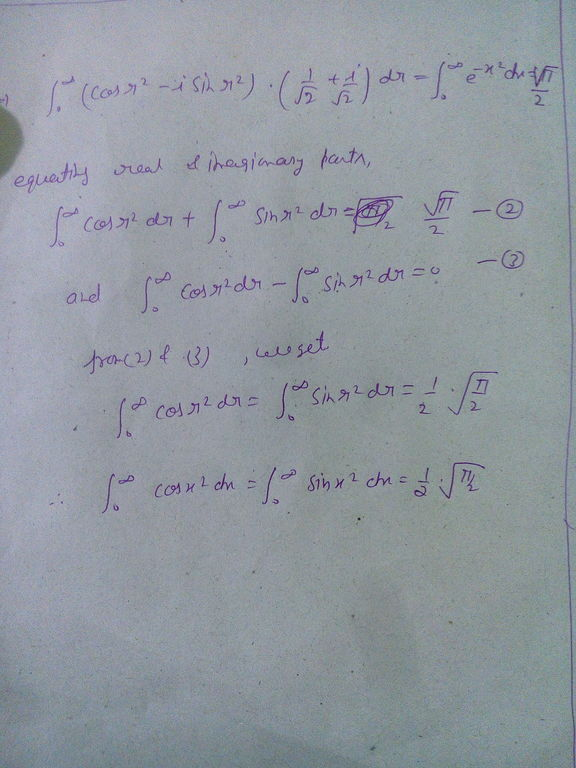# complex analysis onus: Prove that/sin(H)dr=「cos(r2)dr= 0 Hint: Use a closed sector contour as in the previous exercise, but with angle instead. The value of the Gaussian integral will prove usef...

complex analysisonus: Prove that/sin(H)dr=「cos(r2)dr= 0 Hint: Use a closed sector contour as in the previous exercise, but with angle instead. The value of the Gaussian integral will prove useful as well!##### Add Answer of: complex analysis onus: Prove that/sin(H)dr=「cos(r2)dr= 0 Hint: Use a closed sector contour as in the previous exercise, but with angle instead. The value of the Gaussian integral will prove usef...(a) Use complex integration techniques to evaluate the integral Consider a sector contour of the form shown below, whose angle θ may depend on n. As part of your solution, consider that for n 3, if , then the integral on the straight arm of argument can be evaluated using the parametrization z = rei2/3,0 < r < R, to be ežri3 dr dx re (b) Conditions on convergence of rational integrals (i) Find the real number a such that...